# Königsberg bridges: destroying them is the solution

The “Seven Bridges of Königsberg” is a historically notable problem in mathematics:

In the city of Königsberg we have a curious region with 4 land areas (one above, one below and two in the middle) connected by 7 bridges as shown in the figure below.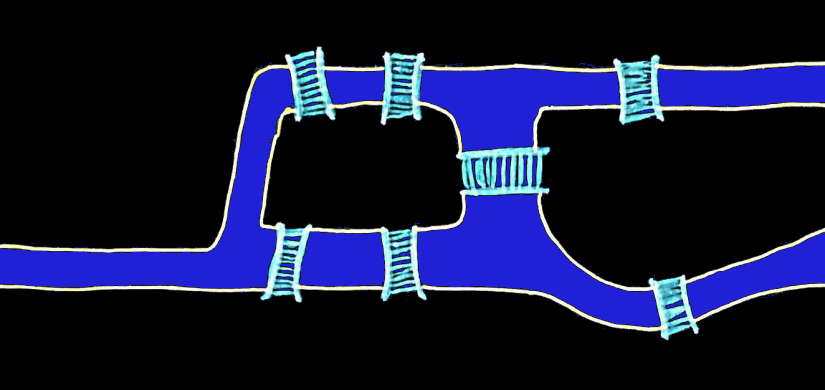Here is the conjecture:

It is possible to start from one of the 4 terra firma areas, go through the 7 bridges and return to the initial area.

Technical details:

1. It is not worth leaving this region of the map;

2. It is not worth crossing the same bridge more than once;

3. It is allowed to cross the river only by crossing the bridges.

Think about this problem before we discuss the resolution. Remember that conjectures are statements that have not yet been proved either as true (in this case they would be theorems) or as false (that is, refuted).

Now that you have thought about this problem for some time, know that the great mathematician Leonhard Euler went through this same dilemma in 1736. As a result of his analysis he came to a resolution that refutes this conjecture (that is, to pass through the Seven Bridges of Königsberg and returning to the original position is impossible). For this, Euler proposed the problem in the following simplified model, in which each circle represents an area of ​​solid land and each line represents a bridge.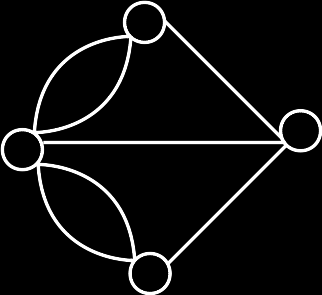In order to understand Euler’s argument, let us call each vertex of land and each bridge edge. Thus, in the representation above, we can see that all vertices are connected by an odd number of edges. This means that if there was a solution, it should start from any vertex and pass through the edges that connect it. But each time it passes through an edge connected to this vertex, we have the following situation:

1. If we were at the apex, then we left it;

2. If we were not at the apex, then we entered it.

As we come out of a vertex, we have to odd that the number of times we pass through edges connected to the vertex, then we will end up outside this vertex. On the other hand, if the number of times that we pass through edges connected to the vertex is even, we return to this vertex.

Note then that in the problem proposed for the Königsberg Bridges, all vertices have an odd number of edges, and the problem asks it to start and end at the same vertex. So, if we go through all the edges, then we go through an odd number of edges connected to that vertex, then we must be outside the vertex. With this, we have demonstrated that there is no solution to this problem.

In this case, paths that make it possible to leave a vertex, traverse all edges only once and return to the original vertex, are called Eulerian graphs. A theorem that follows from this concept is as follows:

A graph will be Eulerian if it is connected and all its vertices have an even number of edges connected to it.

We can understand why they have an even number of edges, because if we pass through the edges of a vertex an even number of times, and we don’t start at that vertex, then we will end up outside that vertex.

Thus, for the famous “Seven Bridges of Königsberg” problem, not finding a solution is different from proving that it does not exist. But as it forms a graph that is not Eulerian, then in fact its solution does not exist. But what about some more chaotic variations? If we happen to destroy one or more bridges, will we have a solution? Next, we will analyze the variations of the problem by destroying its bridges (ignoring variations in symmetry or permutation by isolated vertices).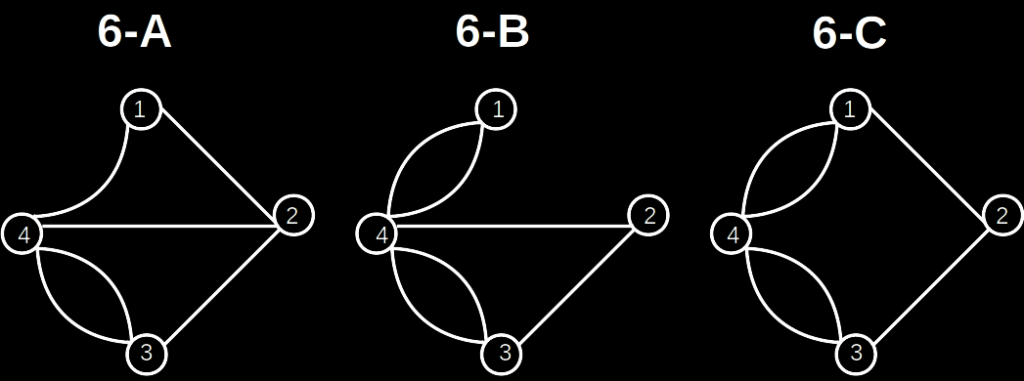6-A. There is no solution, as the vertices 2 and 3 have an odd number of edges.

6-B. There is no solution, as the vertices 3 and 4 have an odd number of edges.

6-C. There is no solution, since vertices 1 and 3 have an odd number of edges.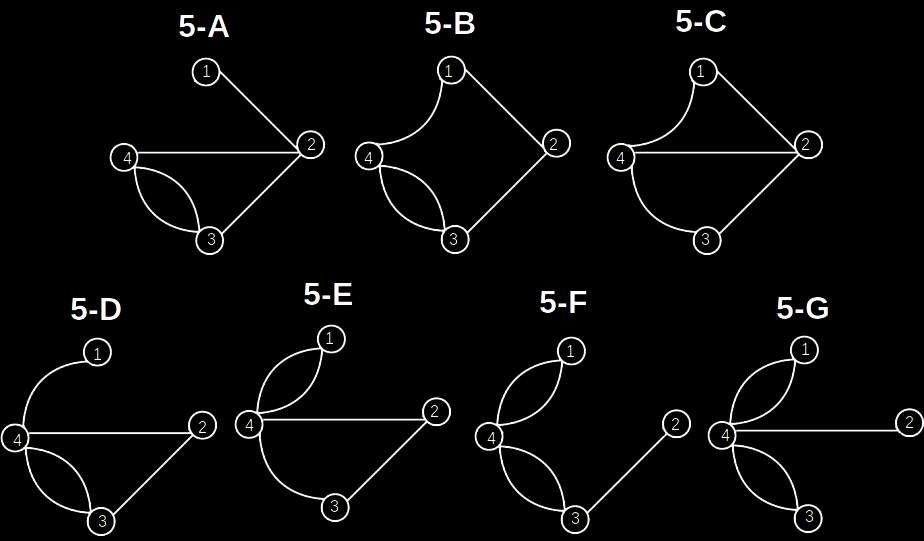5-A. There is no solution, as all vertices have an odd number of edges.

5-B. There is no solution, as the vertices 3 and 4 have an odd number of edges.

5-C. There is no solution, as the vertices 2 and 4 have an odd number of edges.

5-D. There is no solution, since vertices 1 and 3 have an odd number of edges.

5-E. There’s a solution! Start from vertex 1, go to vertex 4, then to vertex 3, then to vertex 2, then to vertex 4, go back to vertex 1.

5-F. There is no solution, as the vertices 2 and 3 have an odd number of edges.

5-G. There is no solution, as the vertices 2 and 4 have an odd number of edges.4-A. There is no solution, since vertices 1 and 2 have an odd number of edges.

4-B. There is no solution, as vertices 1 and 4 have an odd number of edges.

4-C. There is no solution, since vertices 1 and 3 have an odd number of edges.

4-D. There’s a solution! Start from vertex 1, go to vertex 2, then to vertex 3, then to vertex 4, go back to vertex 1.

4-E. There is no solution, as the vertices 3 and 4 have an odd number of edges.

4-F. There’s a solution! Start from vertex 1, go to vertex 4, then to vertex 3, then to vertex 4, go back to vertex 1.3-A. There is no solution, as all vertices have an odd number of edges.

3-B. There is no solution, since vertex 2 has an odd number of edges.

3-C. There is no solution, as the vertices 3 and 4 have an odd number of edges.

3-D. There’s a solution! Start from vertex 1, go to vertex 2, then to vertex 4, go back to vertex 1.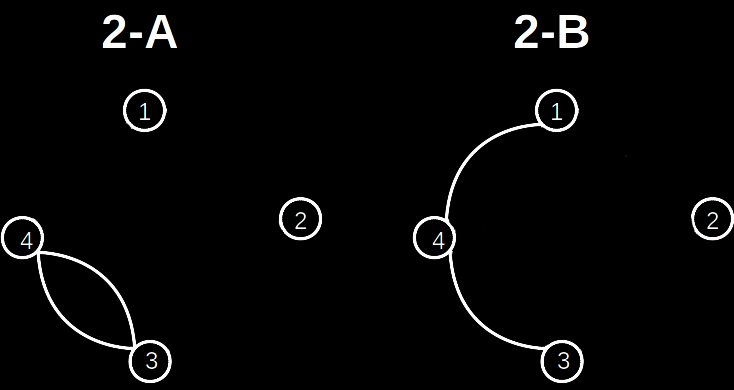2-A. There’s a solution! Start from vertex 4, go to vertex 3, go back to vertex 4.

2-B. There is no solution, since vertices 1 and 3 have an odd number of edges.

The case with 1 bridge is unnecessary, because if there is only one edge, there will be a vertex with an odd number of edges (in this case, 1).

The case with 0 bridges is trivial, because if there are no bridges to cross, we already started at the vertex that satisfies the problem.

With this we can conclude that destroying two or five bridges (or all) the insoluble problem can support solutions, that is, eliminating some specific edges we can transform the original graph into Eulerian graphs.